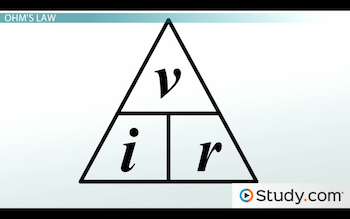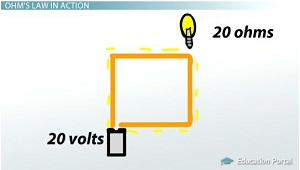# Ohm's Law: Definition & Relationship Between Voltage, Current & Resistance

An error occurred trying to load this video.

Try refreshing the page, or contact customer support.

Coming up next: Electric Circuit Fundamentals: Components & Types

### You're on a roll. Keep up the good work!

Replay
Your next lesson will play in 10 seconds
• 0:08 Pressure, Resistance and Flow
• 1:13 Ohm's Law
• 2:39 Ohm's Law in Action
• 6:26 Lesson Summary

Want to watch this again later?

Timeline
Autoplay
Autoplay
Speed

#### Recommended Lessons and Courses for You

Lesson Transcript
Instructor: Jim Heald

Jim has taught undergraduate engineering courses and has a master's degree in mechanical engineering.

Did you know that electrical current is affected by the voltage and resistance in a circuit? In this lesson, we'll use Ohm's law, which tells us how current, voltage, and resistance are related, as we work through several electric circuit examples.

## Pressure, Resistance, and Flow

This past weekend, I was out in the garden watering the flowers when suddenly the flow of water nearly stopped. I looked behind me and realized there was a kink in the hose that was restricting the water flow. After I straightened out the kink, the water flow came back and I resumed my watering. But, a few minutes later, the water flow slowed down again. I looked for another kink in the hose, but this time I couldn't find one. I went in the house and found that my roommate was taking a shower while running the washing machine and the dishwasher all at the same time! Our water pump couldn't keep up with so much demand for water, and as a result, the water pressure to my garden hose had dropped, which reduced the water flow.

This got me thinking about how similar water flowing through a hose is to electric current in a circuit. Just like the water flow was determined by the amount of pressure and resistance in the hose, electric current is determined by the amount of voltage and resistance in an electric circuit.

## Ohm's Law

The relationship between voltage, current, and resistance is described by Ohm's law. This equation, i = v/r, tells us that the current, i, flowing through a circuit is directly proportional to the voltage, v, and inversely proportional to the resistance, r. In other words, if we increase the voltage, then the current will increase. But, if we increase the resistance, then the current will decrease. We saw these concepts in action with the garden hose. Increasing the pressure caused the flow to increase, but getting a kink in the hose increased the resistance, which caused the flow to decrease.The way the equation is written here, it would be easy to use Ohm's law to figure out the current if we know the voltage and the resistance. But, what if we wanted to solve for the voltage or the resistance instead? One way to do this would be to rearrange the terms of the equation to solve for the other parameters, but there's an easier way. The diagram above will give us the appropriate equation to solve for any unknown parameter without using any algebra. To use this diagram, we simply cover up the parameter we're trying to find to get the proper equation. This will make more sense once we start using it, so let's do some examples.

## Ohm's Law in Action

Below is a simple electric circuit that we'll use to do our examples. Our voltage source is a battery that is connected to a light bulb, which provides resistance to the electric current. To start off with, let's say our battery has a voltage of 10 volts, the light bulb has a resistance of 20 ohms, and we need to figure out the current flowing through the circuit. Using our diagram, we cover up the parameter that we're trying to find, which is current, or i, and that leaves us with the voltage, v, over the resistance, r. In other words, to find the current, we need to divide the voltage by the resistance. Doing the math, 10 volts divided by 20 ohms results in one half ampere of current flowing in the circuit.Next, let's increase the voltage to see what happens to the current. We'll use the same light bulb but switch to a 20-volt battery. Using the same equation as before, we divide 20 volts by 20 ohms and we get 1 amp of current. As we can see, doubling the voltage caused the current to double as well. This makes sense when we think about the garden hose. If we increased the pressure in the hose, we would expect the flow of water to increase as well. It's always good to double-check your work by asking if the results match what you expected to happen.

To unlock this lesson you must be a Study.com Member.

### Register to view this lesson

Are you a student or a teacher?

### Unlock Your Education

#### See for yourself why 30 million people use Study.com

##### Become a Study.com member and start learning now.
Back
What teachers are saying about Study.com

### Earning College Credit

Did you know… We have over 160 college courses that prepare you to earn credit by exam that is accepted by over 1,500 colleges and universities. You can test out of the first two years of college and save thousands off your degree. Anyone can earn credit-by-exam regardless of age or education level.